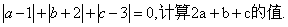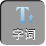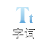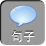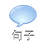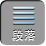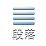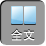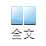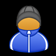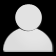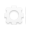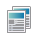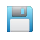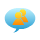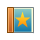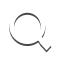-AA+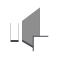1、已知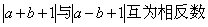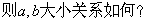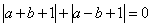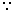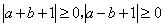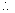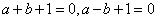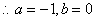a< b

2、化简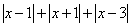.=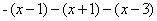=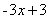-1< x< 1时，= -( x -1)+( x+ 1) -( x -3)= - x+ 5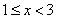时，=（ x -1)+( x+ 1) -( x -3)= x+ 3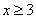时，= x -1+ x+ 1+ x -3= 3 x -3

3、解方程2）整体法：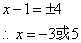3）分析：运用例 2分段讨论的基础

x< 1时，

-x -1) -( x -2)= 4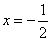;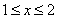时，

x> 2

x= 3.5